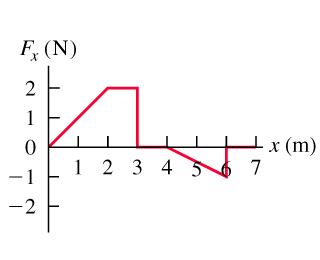# Problem: A force is applied to a 3.5-kg radio-controlled model car parallel to the x-axis as it moves along a straight track. The x-component of the force varies with the x-coordinate of the car as shown in figure .Suppose the model car is initially at rest at x=0 and F is the net force acting on it.Use the work-energy theorem to find the speed of the car at x=3.0 m.Use the work-energy theorem to find the speed of the car at x=4.0 m.Use the work-energy theorem to find the speed of the car at x=7.0 m.

⚠️Our tutors found the solution shown to be helpful for the problem you're searching for. We don't have the exact solution yet.

###### Problem Details

A force is applied to a 3.5-kg radio-controlled model car parallel to the x-axis as it moves along a straight track. The x-component of the force varies with the x-coordinate of the car as shown in figure.Suppose the model car is initially at rest at x=0 and F is the net force acting on it.

Use the work-energy theorem to find the speed of the car at x=3.0 m.

Use the work-energy theorem to find the speed of the car at x=4.0 m.

Use the work-energy theorem to find the speed of the car at x=7.0 m.